# Symbolic execution and smart contracts

Posted on August 7, 2018 by Brian Marick

Posted in

This is the third in a series of posts about the use of the K framework to prove smart contracts correct. The previous post [[[link]]] showed how symbolic execution is used as part of the proving process. This post will show some of the useful things you can do with symbolic execution alone.

## Gas calculations

Symbolic execution can, in some cases, give you an absolute upper bound on how much gas a transaction will use. (Not just an estimate.) In other cases, it can give you a formula that lets you calculate the cost for a particular set of inputs.

We'll continue to use variants of the very simple (but buggy) program from the previous post:

``````function totalSupply() public view returns (uint) {
if (supply == 0x1000000000000000000) {
return 0;
} else {
return supply;
}
}
``````

Since we apply K to compiled code, let's start with a compiled version of the same program: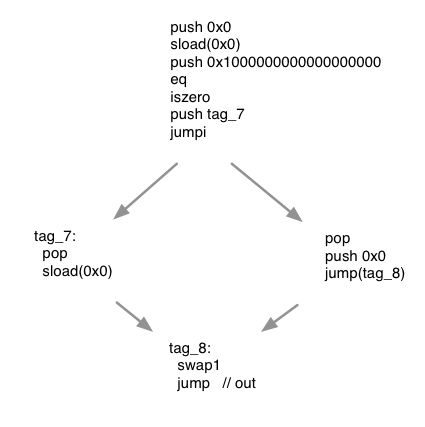Although it's probably not clear from that source, the branch point compares a value, `supply`, from blockchain storage, to a constant (`0x1000000000000000000`). We'll refer to `supply` here as `storage` because that's where the compiler decided to put it.

Gas

When Ethereum smart contracts are executed, they are supplied with a fixed amount of gas to "fuel" their execution. If the calculation runs out of gas, the transaction is cancelled and no results are stored in the blockchain.

Different instructions have different gas costs. `push`, for example, costs 3 gas, but `sload`costs 200. There's also a charge for the maximum amount of non-stack, non-blockchain memory used.

There are two paths from the beginning to the end of the compiled function. We'll emphasize that by duplicating the end segment: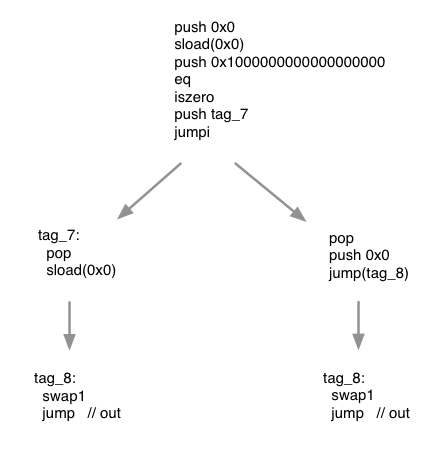The symbolic execution engine will follow both paths until the end of the program. It can, along the way, keep a running total of how much gas each path uses.

The left path costs 438 and the right costs 249. A transaction with 438 gas would never fail.

Loops

A symbolic execution engine can't always give you an exact number. Suppose the function took an array of contract addresses and processed them one by one. The gas cost will depend on the number of addresses, and so be an equation like this:

``````  36833 + 37379 * length_of(addresses)
``````

## Assertions

Symbolic execution can tell when a supposedly impossible condition can in fact be true.

Solidity provides an `assert` statement. The documentation says "Properly functioning code should never reach a failing `assert` statement; if this happens there is a bug in your contract which you should fix."

Let's add an assertion to the end of our function. Because you probably don't want to look at more assembly code, let's use Solidity:

``````uint supply;
function totalSupply() public view returns (uint retval) {
if (supply == 0x1000000000000000000) {
retval = 0;
} else {
retval = supply;
}
assert(retval == supply);
}
``````

`assert` is essentially another `if` statement of this form:

``````if (retval != supply) {
invalid; // fail the transaction. `invalid` is the assembly instruction.
}
``````

We have four paths through the code (since the `assert` comes after both of the original `if` statement's branches). Here they are: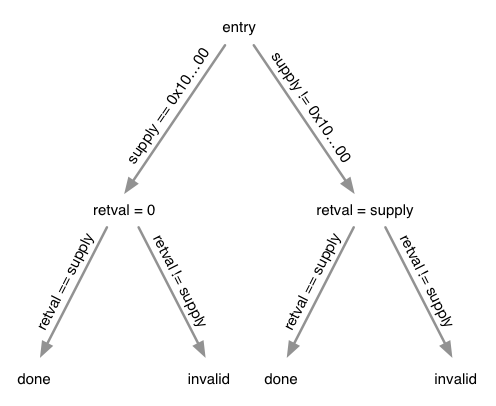Annotations on the branches (like `supply == 0x10...00`) describe what must be true for that branch to be taken. The nodes with assignment statements create new truths; for example, after `retval = supply`, the execution engine knows that `retval` has the same value as `supply` (`retval == supply`).

Let's look at one branch: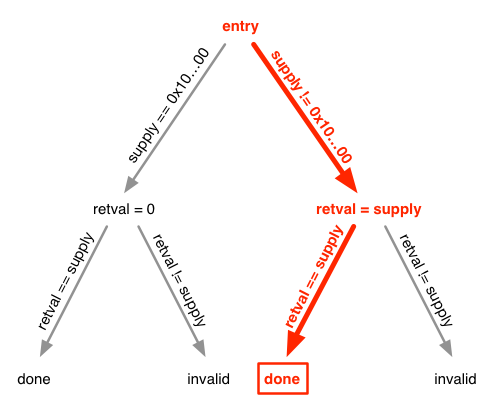At the end of the path, all of these must be true:

• `supply != 0x1000000000000000000`
• `retval == supply` (because of the assignment)
• `retval == supply` (because of the branch annotation)

Can they all be true at the same time? Sure. Whenever `supply` isn't `0x1000000000000000000`.

Here's another branch: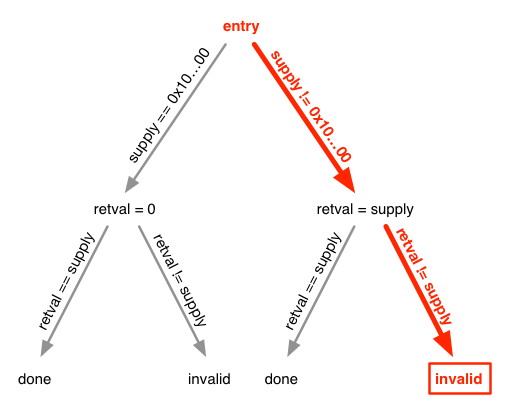Here are the path expressions:

• `supply != 0x1000000000000000000`
• `retval == supply` (because of the assignment)
• `retval != supply` (because of the branch annotation)

The last is new. The last two can't simultaneously be true, so we know the `invalid` is never reached. That is, the assertion will never fail (or "fire") when `supply` is not `0x1000000000000000000`. Which is what we want.

One final path: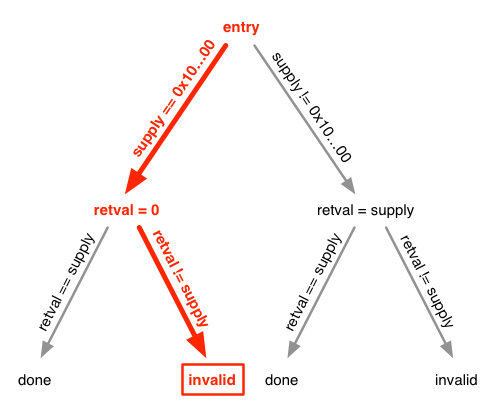Here are the path conditions:

• `supply == 0x1000000000000000000`
• `retval == 0` (because of the assignment)
• `retval != supply` (because of the branch annotation)

If you substitute the known values of `supply` and `retval` into the third expression, you'll see it's true. So `invalid` is reachable. Which means we've found a case that will fire the assertion.

## About that "...you'll see it's true"

Gas calculations are easy because that's just a matter of updating a (possibly symbolic) counter while traversing paths. The "reachability" example required more: what amounts to reducing an equation to either `true` or `false`. In that case, the equation was straightforward: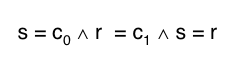(A more comfortable notation for all you mathematicians out there!)

However, it's impossible to write a program that can solve all such equations. And even those it can solve may take a really long time. As with proving (in fact, for the same reasons), the equation solver may need human help to be fast enough.

## The state of the practice

As of this writing, K support for smart contracts only includes gas calculations and calculating whether the (rather small) EVM stack will overflow. There's no support for reachability.

There are other tools based on symbolic execution that can check more things.

Oyente can detect at least the following:

• Failure to check for return values that report an error. (That's implemented by checking that certain function calls are always followed by certain tests.)

• Possible stack overflow.

• Dependence on transaction ordering. This allows (among other things) "front running", in which a contract's owner listens to the network, detects a transaction, and then (with some probability) runs its own transaction first – one that reduces the value of the innocent transaction.

• Vulnerability to an attacker reentering the contract while the contract is running. The famous DAO exploit was a reentrancy attack.

Mythril can [[[blah blah blah. Fill in if decide to go this route.]]]

The difference between these tools and our approach is that we build from a complete semantics of the EVM virtual machine. Their semantics are customized to the particular checks they make. We are serenely confident that a complete semantics, while more time-consuming to develop, will pay off by making it easier to add new checks.The tortoise and the hare image is from Pixabay and is CC0 licensed free for commercial use